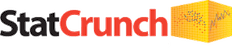# StatCrunch Tutorial VideosStatCrunch is a great statistical analysis app that resides on the web and thus works equally well for both PC and MAC users. If you are taking a stats course and do not have good Excel skills, I suggest you consider learning to use StatCrunch. My sense is that students find StatCrunch’s learning curve, the time and effort needed to get up to a reasonable skill level, is much shorter and not as steep as learning both basic Excel skills and how to use Excel’s statistical formulas to crunch the numbers. This is especially true for short time frame, i.e. 8-week term, introductory statistics classes.

StatCrunch homepage If you bought access to MyStatLab, which has StatCrunch embedded, you should be able to use your Pearson MySTatLab to log in here. If you no longer have access to MyStatLab, you can purchase a 6 or 12 month access key for \$13.75/\$24.20.

StatCrunch Examples: If you prefer written instructions, this page contains links to over 50 step-by-step instructions for most of the topics covered in this course.

StatCrunch playlist on YouTube A list of 93 StatCrunch video tutorials.

StatCrunch: Getting Started – Full Length 7:56-minutes on loading an external data file, calculating summary statistics and creating a simple column chart.

Note: if you are in MyStatLab, you may be able to load problem data directly into StatCrunch by clicking on the table icon as shown below:Videos 2 through 18 in the playlist are relatively short and to the point of the topic in the video title, mainly how to create various charts and tables. The remaining videos are also relatively short and to the point.

Other videos:

Using StatCrunch to help with homework problems in MyLabsPlus (MyStatLab) 8:45 video showing the basics of using StatCrunch in MyStatLab. Includes creating a relative frequency histogram

A 4:18 video showing how to calculate factorials, permutations, and combinations using the StatCrunch compute tool.

13-minute video showing how to use the StatCrunch calculators to determine critical values for the Z, t, Chi-Square, and F tests.

StatCrunch – Calculators for P-Values and Distribution Critical Values A 11:22 video showing how to get both critical values given an alpha and how to get p-values given a test statistic.

Solve Conditional Probabilities use StatCrunch A 4:20 video showing how to use a contingency table in StatCrunch to solve a conditional probability problem.

Probability Problem for Sample Mean using StatCrunch This 5:42-minute video shows the wrong way and the right way to determine the probability that a sample mean is different from a population mean using StatCrunch normal probability calculator.

Find Z-stat and p-value of a test of a Mean using StatCrunch. A 4:47-minute video re solving for the test statistic and probability for a hypothesis test of a claim re a sample mean.

A 2:20 video finding the minimum sample size for a given confidence level and margin of error.

Confidence Interval for the Difference between Two Proportions using StatCrunch A 5:32-minute example.

Hypothesis Testing with StatCrunch A 5:14-minute video on a hypothesis test for a proportion. Note: this is for an older version of StatCrunch but the process is essentially the same.

Hypothesis Test for a Proportion Using StatCrunch an 8:59-minute video on a test for a proportion problem (not in MYSTATLAB but similar).

42 minute video showing how to use StatCrunch to solve a MyStatLab regression and prediction problem.

5 by 3 Contingency Table Created using StatCrunch 3:36 minute showing how to create a contingency table that could be used to solve conditional probability questions or a Chi-Square problem.

Two that may be particularly helpful in the Chi-Square portion of the course are videos 10 and 11 which show how to create contingency tables from both raw data and summary data. On the resulting table, StatCrunch also includes the results of a Chi-Square hypothesis test for independence.

A 6:36-minute video of an example problem from MyStatLab requiring a 2 by 3 Chi-Square test for independence.

StatCrunch – Chi Square Goodness of Fit Example a 2:22- minute video of a Chi-Square goodness of fit test on a problem from MYSTATLAB.

StatCrunch Chi-Squared Goodness of Fit Another 3:23 MYSTATLAB example Goodness of Fit/ Chi-Square problem.

This site uses Akismet to reduce spam. Learn how your comment data is processed.